# 20 Must Know HSC Chemistry Questions with Solutions

Here are 20 must-know HSC Chemistry questions with solutions Learnable's HSC Chemistry author predicts for your upcoming HSC Chemistry Exam.

## Looking for HSC Chemistry practice questions?

Learnable’s HSC Chemistry author shares their compilation of 20 HSC Chemistry questions you should know for the HSC chemistry exam. The questions consist of exam-style questions on the four modules:

• Module 5: Equilibrium and Acid Reactions
• Module 6: Acid / Base Reactions
• Module 7: Organic Chemistry
• Module 8: Applying Chemical Ideas

## New HSC Chemistry Questions on Module 5 ‘Equilibrium and Acid Reactions’

### Question 1: Concentration Profile Diagram

Consider the following diagram for the equilibrium:

N2O4(g) $\leftrightharpoons$  2NO2(g)                     $\Delta$H = + 57 kJmol-1(a) Identify the stress applied at time t2. Explain your answer. 3 marks (b) At time t3 the volume of the reaction vessel was reduced.Sketch how the concentrations would change from t3 2 marks (c) Explain the changes shown on your graph 2 marks

See Question 1 Solution

### Question 2: Equilibrium Constant Calculation

2.00 moles of hydrogen gas and 7.00 moles of fluorine gas are mixed in a 3.00 L container, and react to form hydrogen fluoride.

H2(g)   + F2(g)   ⇌ 2HF(g)

The equilibrium constant for this reaction is 1.15 × 102 at 298K. Calculate the pressure inside the flask at equilibrium. (4 marks)

See Question 2 Solution

### Question 3: Ionic Compounds in Water

Describe the processes occurring when solid sodium sulfate dissolves exothermically in water in terms of:

 (a) intramolecular forces 3 marks (b) entropy 2 marks (c) enthalpy 1 mark

See Question 3 Solution

### Question 4: The Common Ion Effect

What is the solubility of lead (II) bromide, in molL-1, in 0.20M sodium bromide solution? (3 marks)

See Question 4 Solution

### Question 5: Ksp Calculations

What volume of water is required to dissolve 8.5 kg of magnesium hydroxide? (3 marks)

See Question 5 Solution

## New HSC Chemistry Questions on Module 6 ‘Acid / Base Reactions’

### Question 6:  Acid Definitions

Explain why 0.1 M solutions of sulfuric acid, acetic acid and hydrochloric acid have different pH values. (3 marks)

See Question 6 Solution

### Question 7: Acidic and Basic Salts

Explain why the equivalence point of a titration between sodium hydroxide and acetic acid is not neutral. Include chemical equations in your answer. (3 marks)

See Question 7 Solution

### Question 8: Calculating pH after Dilution

10.0 mL of a solution of acetic acid at pH 2.13 was diluted to 100.0mL. What is the pH of the new solution? The pKa of acetic acid is 4.75.  (4 marks)

See Question 8 Solution

### Question 9: Titration Technique

Describe AND justify at least four steps you took in performing an analysis of acid concentration to reduce experimental errors and thus ensure your titration results were valid and reliable. (4 marks)

See Question 9 Solution

### Question 10: Titration Curves and Conductivity Graphs

Sally performs the following titrations:

 Titration Conical Flask Burette X KOH HF Y HBr NaOH Z HNO3 NH3

 (a) Which titration(s) would produce curves that display a buffering region 1 mark (b) Explain why the buffering region arises in a titration you have identified above. Include a balanced chemical equation in your answer. 3 marks (c) Sketch the shape of the expected titration curve for titration Z. Label the equivalence point 2 marks (d) Sketch the shape of the expected conductivity graph for titration Z. Label the equivalence point 2 marks

See Question 10 Solution

## New HSC Chemistry Questions on Module 7 ‘Organic Chemistry’

### Question 11: Physical Properties of Organic Substances

Account for the usefulness of ethanol as a solvent in terms of its structure. Refer to a diagram in your answer (4 marks)

See Question 11 Solution

### Question 12: Biofuels

Bioethanol and biodiesel are able to be used in cars as alternatives to petrol and diesel. Evaluate the sucess of the current usage of bioethanol and biodiesel as car fuels. (5 marks)

See Question 12 Solution

### Question 13: Enthalpy of Combustion

The heat of combustion of ethanol is 1367 kJmol-1. A mass of ethanol undergoes complete combustion to release 2320 kJ of heat. What mass of carbon dioxide is formed? (3 marks)

See Question 13 Solution

### Question 14: Reaction Pathways

Examine the reaction pathway below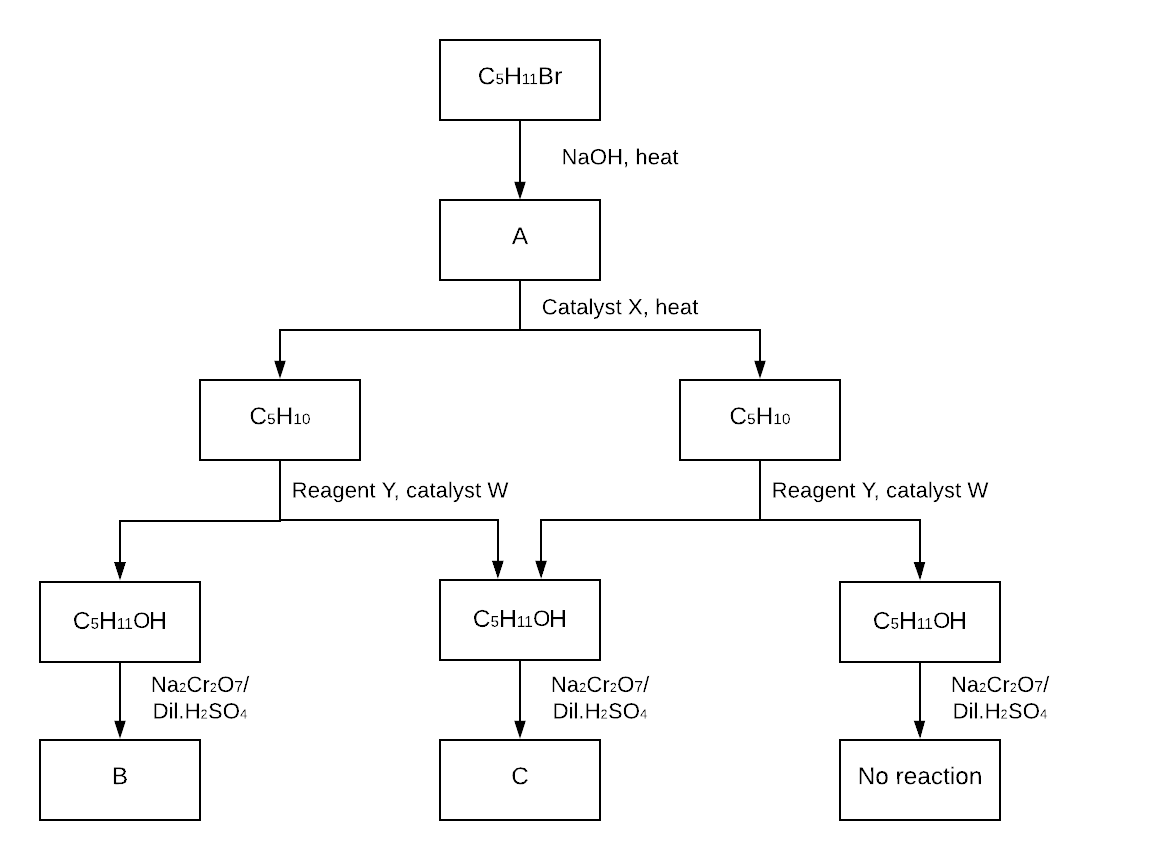(a) Identify the missing reagents and catalyst that are required for each of the transformation. 2 marks (b) Draw the structures of the compounds A to C, justifying your diagrams with reference to the conditions provided. 3 marks

See Question 14 Solution

### Question 15: Polymers

 (a) Compare the production of polyethylene and polyethylene terephthalate from their component monomers. Include relevant chemical equations in your answer. 4 marks (b) Account for the uses of each polymer above, in terms of their structures and properties. 4 marks

See Question 15 Solution

## New HSC Chemistry Questions on Module 8 ‘Applying Chemical Ideas’

### Question 16: Ion Identification

To identify the three anions present in a solution, the following procedure was performed: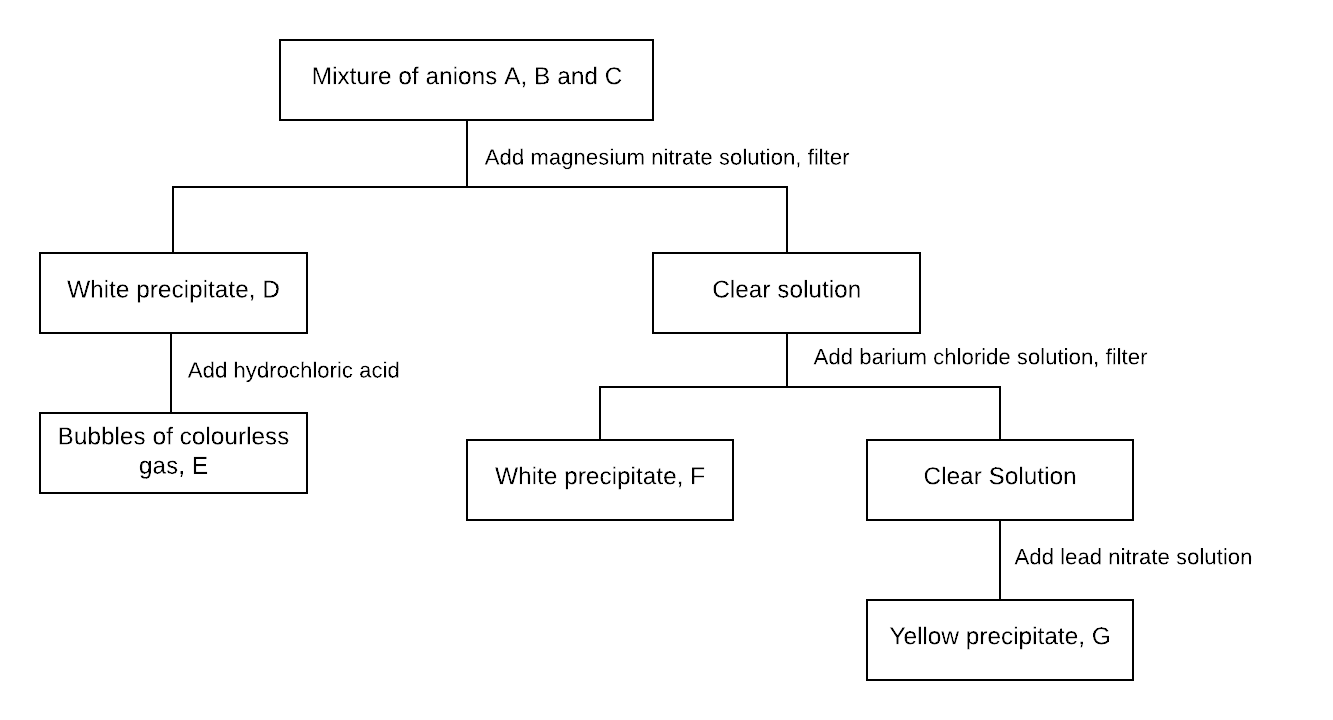(a) Identify precipitate D and gas E 2 marks (b) Write net ionic equations for the formation of precipitates F and G 2 marks (c) A compound is known to be either calcium chloride or iron(III) chloride. Describe a simple test that could be used to distinguish between the two. 1 mark

See Question 16 Solution

### Question 17: Mass Spectroscopy

The mass spectrum of chloroethane contains peaks at m/z = 29, 64 and 66.

 (a) Draw the structure of the fragment producing the peak at m/z = 29. 1 mark (b) Write a balanced equation to show the formation of the fragment identified in (a) from the molecular ion. 1 mark (c) Explain how the two peaks at m/z = 64 and 66 arise, and predict their relative heights. 3 marks

See Question 17 Solution

### Question 18: NMR Spectroscopy

 (a) State the type of radiation used in NMR spectroscopy 1 mark (b) Explain why carbon-12 nuclei cannot be used in NMR spectroscopy 1 mark (c) Explain the function of the magnet in an NMR spectrometer 2 marks

See Question 18 Solution

### Question 19: Colourimetry

A coordination complex is formed when Ni2+ reacts with a ligand X according to the following equation:

aNi2+(aq)   +    bX(aq)    →    [Nia(X)b]2a+(aq)

Where a and b are numbers representing the ratio in which Ni2+ and X combine.

Eight 10mL samples containing different ratios of Ni2+ and X were prepared and analysed using spectrophotometry to determine the stoichiometry of the reaction. The absorbance of the samples is tabulated below.

 Samples Volume of Ni2+ solution (mL) 0.00 1.00 2.00 3.00 4.00 5.00 6.00 7.00 10.00 Volume of X solution (mL) 10.00 9.00 8.00 7.00 6.00 5.00 4.00 3.00 0.00 Absorbance at 628 nm 0.00 0.22 0.44 0.66 0.88 0.84 0.76 0.00 0.00

 (a) On the grid below, construct a graph of absorbance against volume of Ni2+ solution from 0.00 mL to 6.00 mL, and draw TWO lines of best fit.3 marks (b) From the lines of best fit, determine the maximum absorbance of the complex and the corresponding volumes of Ni2+ and X–. 1 mark (c) The solution of Ni2+ used in this investigation was prepared using 6.56g of nickel(II) chloride hexahydrate dissolved in 100.0 mL of distilled water. The solution of the ligand X had a concentration of 0.100 M. Calculate the number of moles of each reagent corresponding to the maximum absorbance and hence the formula of the complex. 3 marks

See Question 19 Solution

### Question 20: Designing Reaction Synthesis Process

Sodium carbonate can be produced industrially via Solvay process. The overall reaction of Solvay process is shown below.

2NaCl(aq) + CaCO3(s) → Na2CO3(s) + CaCl2(aq)

Outline two reasons why the Solvay process plant may be located near the ocean, and justify your answer. (4 marks)

See Question 20 Solution

## Solutions to HSC Chemistry Questions 1 – 20

1(a) At t2 there is a decrease in the concentration of products (NO2) and an increase in reactants (N2O4) with no spikes in concentrations, thus the equilibrium shifted to the left, in the exothermic direction, when temperature was changed. According to Le Chatelier’s principle, a decrease in temperature will cause the equilibrium to shift to the exothermic side to produce more heat and minimise the disturbance. Therefore the stress at time t2 is a decrease in temperature.

(c) Decreasing volume causes the concentration of all components to increase (spike up in graph). According to Le Chatelier’s principle, the position of equilibrium will shift to the side with less gas moles (left), to decrease the pressure and minimise the disturbance. This decreases the concentration of NO2 and increases the concentration of N2O4. Eventually, the rates of the forward and reverse become equal and the concentrations remain constant.

Back to Question 1

2Total moles of gas = 9.00 mol

$P = \frac{nRT}{V} = \frac{9.00 × 8.314 × 298}{3.00} = 7430 kPa (3 s.f.)$

Note: Since there are two moles of gas on both sides, there will be no change in pressure when the system reaches equilibrium compared to the system initially. Hence the pressure at equilibrium remains as 7430 kPa.

Back to Question 2

3(a) Ionic bonds in sodium sulfate and hydrogen bonds in water break as the ions begin to dissolve. Ion-dipole forces form between individual ions and water, arranging the water molecules into solvation layers around the ions.

(b) The entropy of the solute increases as sodium sulfate dissolves because the dissociated ions are more dispersed. The entropy of the solvent decreases due to the formation of an organised solvation sphere of water molecules around the solute.

(c) Since the dissolution of sodium sulfate in water is exothermic, the enthalpy decreases as sodium sulfate dissolves.

Back to Question 3

4Step 1: Write a balanced chemical equation for the dissolution of the lead(II) bromide. Don’t forget to include states!

PbBr2(s)  ⇌ Pb2+(aq)  + 2Br(aq)

Step 2: Write the corresponding equilibrium constant expression

Ksp = [Pb2+][Br]2 = 6.60 x 10-6

Step 3: Find the initial concentration of the common ion

NaBr(s)  → Na+(aq)  + Br(aq)

[Br]initial = 0.2 M

Step 4: Construct an ICE table and calculate the equilibrium concentration of all substances in terms of 𝑥 by making an appropriate assumption

Let the moles of PbBr2 that dissolve in 1 L be 𝑥

 PbBr2(s) Pb2+(aq) 2Br–(aq) Initial concentration excess 0 0.2 Change in concentration – 𝑥 + 𝑥 + 2𝑥 Equilibrium concentration excess – 𝑥 𝑥 0.2 + 2𝑥

Assume that 2𝑥 is very small compared to [Br-] initial, therefore 0.2 + 2𝑥 ≈ 0.2

Step 5: Substitute the equilibrium concentrations and the given value of Ksp into the equilibrium constant expression and solve for 𝑥.

$K_{sp} = [Pb^{2+}][Br^-]^2$

$6.60 \times 10^{-6} = x \times 0.2^2$

$x = \frac{6.60 \times 10^{-6}}{0.2^2}$

$x = 1.65 \times 10^{-4}$

Therefore [PbBr2] in the final solution is 1.7 x 10-4 M (2 s.f.)

Back to Question 4

5 Step 1: Write a balanced chemical equation for the dissolution of magnesium hydroxide

Mg(OH)2(s)  ⇌ Mg2+(aq) +  2OH(aq)

Step 2: Write the corresponding equilibrium constant expression

Ksp = [Mg2+][OH]2 = 5.61 x 10-12

Step 3: Calculate the ion concentrations in terms of 𝑥

Let moles of Mg(OH)2 that dissolves in 1 L = 𝑥, thus [Mg2+] = 𝑥, [OH] = 2𝑥

Step 4: Substitute into the equilibrium constant expression and solve for 𝑥

Ksp =  (𝑥)(2𝑥)2 = 4𝑥3

5.61 × 10-12 = 4𝑥3

𝑥 = 1.119 × 10-4

Solubility of Mg(OH)2 = 1.119 × 10-4 mol/L

Step 5: Convert solubility to the required units

m(Mg(OH)2) in 1 L = n × MM

= 1.119 × 10-4 × (24.31 + 16.00 × 2 + 1.008 × 2)

= 6.5287 × 10-3 g

= 6.5287 × 10-6 kg

Litres required to dissolve

$8.5 kg = \frac{8.5}{6.5287\times10^{-6}} = 1.3 \times 10^6 \ L\ (2\ s.f.)$

Back to Question 5

6HCl is a strong monoprotic acid which completely ionises in water:

HCl(aq) → H+(aq) + Cl(aq)

Hence the concentration of H+ in 0.1 M HCl will be 0.1 M and pH will be 1.

Acetic acid is a weak monoprotic acid which doesn’t completely ionise in water: CH3COOH(aq) ⇌ CH3COO(aq) + H+(aq)

Hence the concentration of H+ will be less than 0.1 M and pH will be > 1.

H2SO4 is a strong diprotic acid which completely ionises in water:

H2SO4(aq) → 2H+(aq) + SO42-(aq)

Hence the concentration of H+ will be 0.2 M and pH will be 0.7.

Back to Question 6

7Sodium hydroxide and acetic acid react as shown:

CH3COOH(aq)  + NaOH(aq) →  NaCH3COO(aq) + H2O(l)

At equivalence point, only NaCH3COO and water are present.

NaCH3COO is dissociated into sodium and acetate ions. Acetate is basic as it can accept H+ from water, producing OH and therefore a basic equivalence point:

CH3COO(aq)  + H2O(l) ⇌ CH3COOH(aq)  + OH(aq)

Back to Question 7

8CH3COOH(aq)  ⇌ H+(aq) + CH3COO(aq)

$K_a = \frac{[H^+][CH_3COO^-]}{CH_3COOH}=10^{-4.75}$

Working out concentration:

 CH3COOH H+ CH3COO– Initial concentration C 0 0 Change in concentration – 10-2.13 + 10-2.13 + 10-2.13 Equilibrium concentration C – 10-2.13 10-2.13 10-2.13

Ka = $\frac{(10^{-2.13})^2}{C - 10^{-2.13}}$ = 10-4.75

(10-2.13)2 = 10-4.75 × (C – 10-2.13)

$\frac{(10^{-2.13})^2}{10^{-4.75}}$ = C – 10-2.13

C = 10-2.13 + $\frac{(10^{-2.13})^2}{10^{-4.75}}$ = 3.0977 M

Dilution

c1v1 = c2v2

c2 = 0.30977 M

pH of diluted solution:

 CH3COOH H+ CH3COO– Initial concentration 0.30977 0 0 Change in concentration –𝑥 +𝑥 +𝑥 Equilibrium concentration 0.30977 – 𝑥 𝑥 𝑥

Since 𝑥 is very small, 0.30977 – 𝑥 ≈ 0.30977

$K_a = \frac{x^2}{0.309077}=10^{-4.75}$

𝑥 = 2.347 x 10-3 = [H+]

pH = 2.6295 = 2.63 (2 s.f.)

Back to Question 8

• Rinse the pipette with the filling solution three times, to ensure that the correct concentration is transferred
• Rinse the burette with the filling solution three times, to ensure that the correct concentration is added during the titration
• Take burette readings with the meniscus at eye level to prevent parallax error
• Commence the titration with the liquid level at 0–5 mL to improve reproducibility
• Use an appropriate indicator to determine the equivalence point accurately
• Add a few drops of indicator only as it is a weak acid or base, and will change the titres
• Repeat the experiment to improve reliability

Back to Question 9

10(a) X and Z

(b) Titration Z: HNO3(aq)  + NH3(aq) → NH4NO3(aq)

In this titration, when an excess of NH3 (weak base) is present, NH4+ (its conjugate acid) is also present, forming an equilibrium that can absorb excess H+ or OH. This stabilises pH, hence forming a buffering region where the pH changes slowly.

(c)

##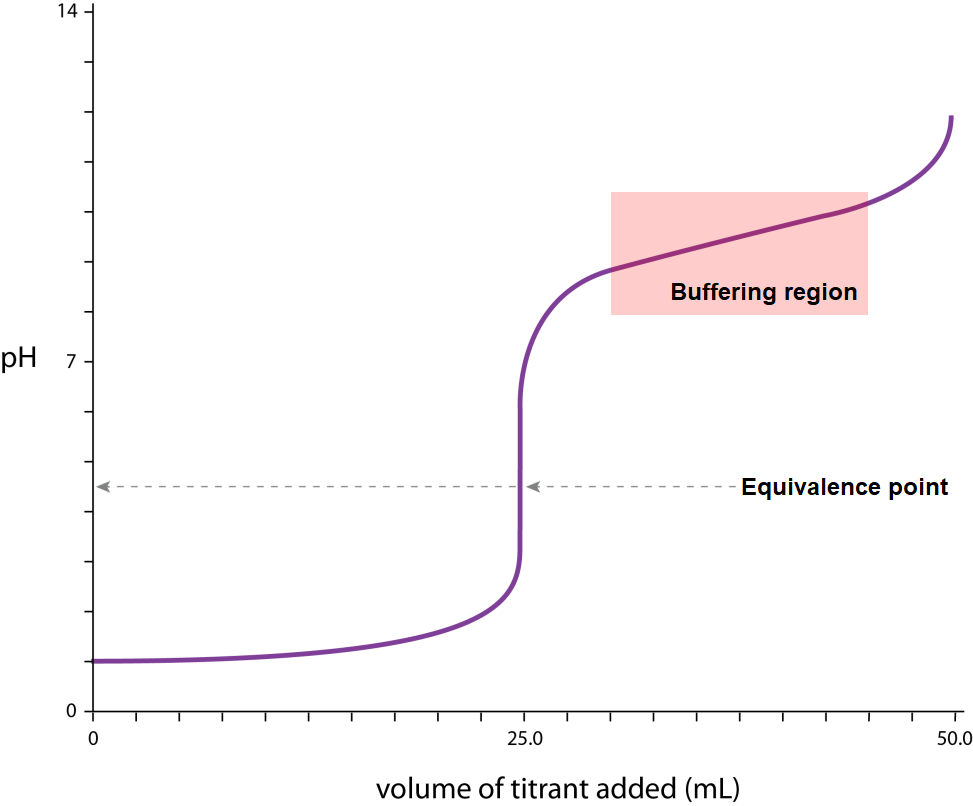(d)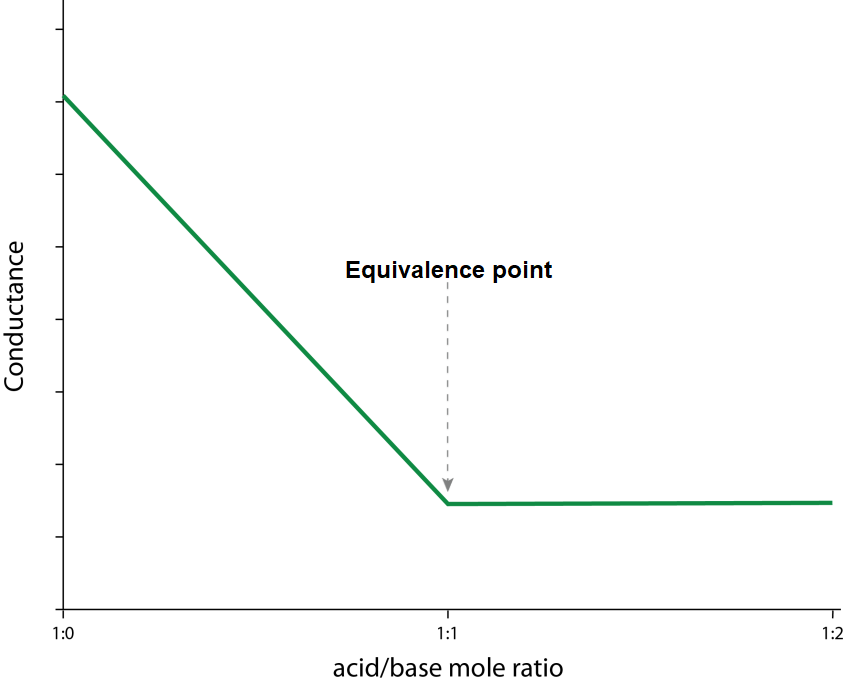Back to Question 10

11Ethanol is a small molecule that contains both a polar and non-polar portion. The OH end can form adhesive hydrogen bonds and dipole-dipole interactions with polar solutes, while the non-polar carbon chain end can form adhesive dispersion forces with non-polar solutes. This means the adhesive forces will be stronger than the cohesive forces, causing dissolution. This makes ethanol an extremely good solvent for both polar and non-polar substances.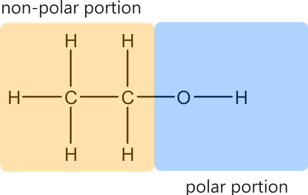Back to Question 11

12 The current use of bioethanol and biodiesel as a fuel source has an overall positive affect on the environment.

Bioethanol
Currently, up to 10% of bioethanol (E-10) can be used in cars without engine modification. This use of bioethanol is beneficial to the environment as ethanol is a much cleaner burning fuel than petrol which consists primarily of octane. Therefore the combustion of bioethanol produces less C(s) and poisonous CO(g). Additionally, the removal of CO2 from the atmosphere during the production of the biomass used in bioethanol production reduces the net release of CO2 into the atmosphere. Currently, bioethanol is mainly produced from waste products such as molasses so the current production and use of bioethanol does not require large amounts of arable land. Thus, the current use of bioethanol is advantageous.

Biodiesel
Biodiesel can be used up to 20% concentrations without engine modification. The use of biodiesel similarly reduces the net release of CO2 into the environment. Since biodiesel is currently produced from waste products (eg. waste oil from restaurants), it has no significant negative environmental impact. Thus, the current use of biodiesel is similarly advantageous.

Back to Question 12

13Step 1: Write a balanced chemical equation for the combustion of ethanol

C2H5OH(l) + 3O2(g) → 2CO2(g) + 3H2O(l)

Step 2: Calculate the number of moles of ethanol that was consumed in the reaction

n(C2H5OH) = -q/H

= 2320/1367

= 1.697147 mol

Step 3: Using stoichiometry calculate the moles of carbon dioxide released

n(CO2) = n(C2H5OH) × 2

= 3.394294 mol

Step 4: Convert moles to mass

m(CO2) = 3.394294 × (12.01 + 2 × 16) = 149.4 g (4 s.f.)

Back to Question 13

14(a) X: Conc. H2SO4, Y: water, W: dil. H2SO4

(b) The alcohol at the bottom right is tertiary as it does not react with dichromate. Therefore it is 2-methylbutan-2-ol (the only saturated tertiary alcohol with 5 carbons).

The alcohol above C is either 2-methylbutan-1-ol or 3-methylbutan-2-ol. Since it can be formed from the hydration of two different alkenes using dilute H2SO4 and water, it can’t be 2-methylbutan-1-ol. Therefore it is 3-methylbutan-2-ol, and it can be oxidised with dichromate to form C, which is 3-methylbutan-2-one.

This means the alkene on the right side must be 2-methylbut-2-ene.

The other alkene that can be hydrated under the same conditions to produce 3-methylbutan-2-ol is 3-methylbut-1-ene. This means that the alcohol above B is 3-methylbutan-1-ol, and it can be oxidised with dichromate to B, 3-methylbutanoic acid.

A dehydrates with concentrated H2SO4 to form 2-methylbut-2-ene and 3-methylbut-1-ene, therefore it is 3-methylbutan-2-ol.

##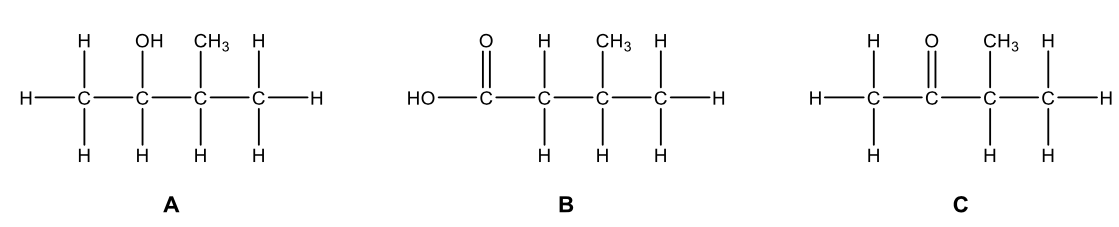Back to Question 14

15(a) Polyethylene

Monomer: ethylene (one monomer only)

Type of reaction: Addition polymerisation, involves the stepwise addition of unsaturated monomers without the elimination of any atoms

Equation: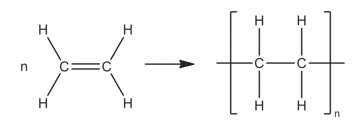Polyethylene terephthalate

Monomers: terephthalic acid, ethylene glycol (two monomers form a copolymer)

Type of reaction: Condensation polymerisation, involves reaction of difunctionalmonomers which eliminates a small molecule (water)

Equation: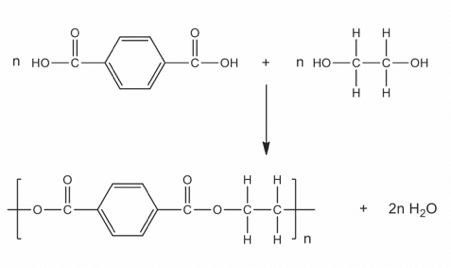(b) Polyethylene (LDPE): cling film, squeezy bottles

LDPE consists of long hydrocarbon chains with significant branching. The branches prevent close packing of the polymer chains, resulting in a low-density plastic that is softer and has a lower melting point, useful for making cling film, more flexible toys and plastic bottles.

PET: clothing fabric, drink bottles

The benzene ring in PET leads to chain stiffness. The polymer chains are stiff and do not deform easily, which gives PET clothing the ability to resist wrinkles and drink bottles the ability to resist impact.

PET can be processed to an amorphous form, which allows light to pass through it (transparence) for drink bottles.

Back to Question 15

16(a) D = magnesium carbonate, E = carbon dioxide

(b) F: Ba2+(aq) + SO42-(aq) → BaSO4(s)

G: Pb2+(aq) + 2l(aq) → PbI2(s)

(c) Add sodium hydroxide solution to the compound. If a white precipitate forms it is calcium chloride, if a brown precipitate forms it is iron(III) chloride (other answers possible).

Back to Question 16

17(a)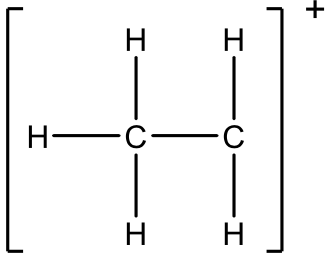(b) C2H5Cl+(g)  → C2H5+(g) +  Cl(g)

(c) The two peaks come from the two common isotopes of chlorine, 35Cl and 37Cl. m/z = 64 corresponds to C2H535Cl+ while m/z = 66 corresponds to C2H537Cl+. Due to their relative abundances, the ratio of heights will be 3:1.

Back to Question 17

(b) They are not spin-active as they contain an even number of protons and an even number of neutrons (6 of each)

(c) The magnet aligns the spins to create two energy levels (parallel and antiparallel), so the nuclei can absorb radiation

Back to Question 18

19(a)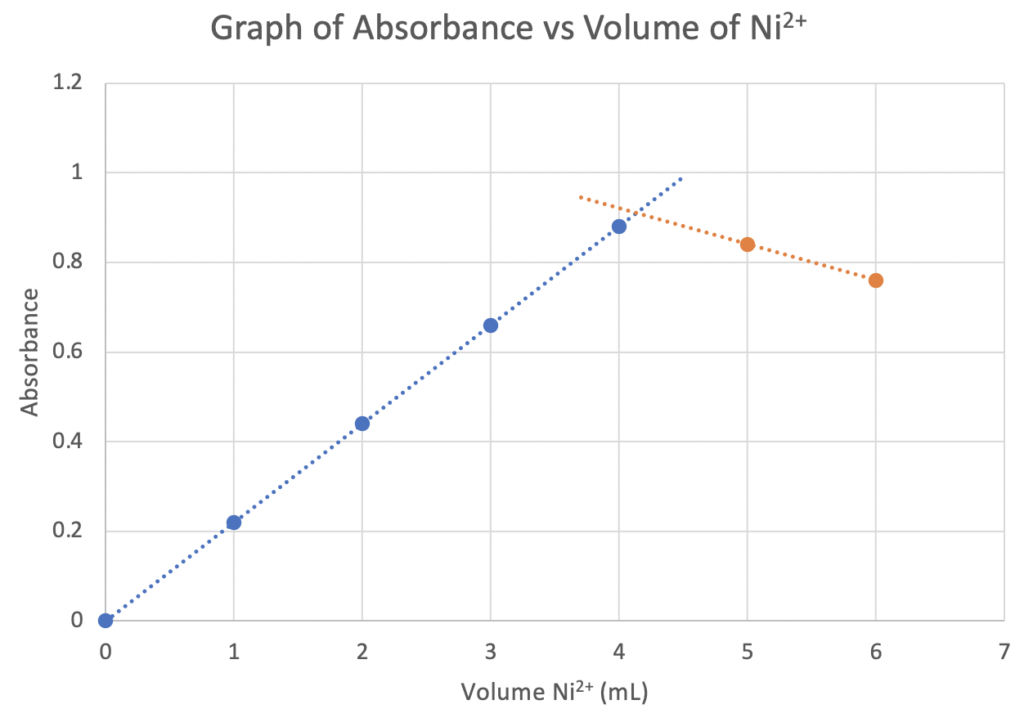(b) A maximum absorbance of 0.92 occurs at a volume of 4.2 mL of Ni2+ and 5.8 mL of X(c) nNi2+$\frac{6.56g}{237.69 gmol^{-1}}$ = 0.02759897345 mol

cNi2+ = $\frac{0.02759897345 mol}{0.1000 L}$ = 0.2759897345 mol L-1

Calculate the moles of Ni2+ at the max absorbance

n(Ni2+) = 0.275989735 mol L-1 × 4.2 × 10-3

= 0.00115915688 mol

Calculate the moles of X at max absorbance

n(X) = 0.100 mol L-1 × 5.8 × 10-3 L

= 0.00058 mol

Ratio of Ni2+ : X = 0.00115915688 mol : 0.00058 mol

= 1.99854 : 1

= 2 : 1

Therefore, the complex is [Ni2(X)]4+.

Back to Question 19

20Reactant availability: Brine (concentrated salt solution), can be sourced from seawater, so a coastal location would allow ready access to this reactant.

Proximity to import and export routes: The other reagents can be easily imported by sea. The product (sodium carbonate) can be exported easily as well.

Availability of cooling water: Cooling of the mixture from the reaction vessel is required. This can be done using sea water as a cheap source.

Calcium chloride disposal: Calcium chloride is non-toxic and soluble. It can be safely and cheaply dumped into the sea since the sea is already rich in calcium and chloride ions.

Back to Question 20

## Access over 1000+ HSC Chemistry Exam-Style Questions on Learnable.

Test your understanding of any HSC Chemistry concepts with Learnable’s customisable quizzes. Try Learnable for free now.

• Instant feedback on syllabus specific questions
• Step-by-step detailed solutions
• Intelligent reporting### Written by Hee-Chan Jang

Hee-Chan is the author of Chemistry resources on Learnable. He loves teaching and helping students to "learn smarter", using his multidisciplinary knowledge of science and engineering. He is also currently completing his doctoral degree in mineral processing at The University of Sydney.